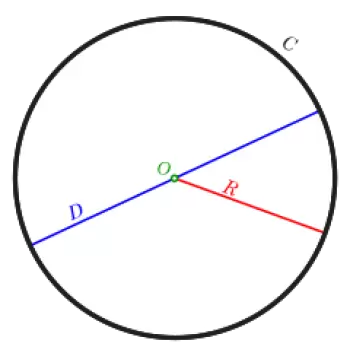# What Is a Circle? Parts and Definition of a CircleA circle is a plane geometric shape whose points are equidistant from a given point on that plane (the center). The distance of the circumference points to the center is the radius or radius. The word circle is derived from a Greek word meaning 'hoop OR ring.'

Never confuse a circle with a polygon. It is not a polygon because it is made up of curves.

The history of this shape is ancient. People used to believe that the moon, sun, and other planets were circular because there was no concept of three-dimensional shapes; mathematicians studied circles that helped them develop calculus and astronomy.

Congruent circles have the same radius, regardless of where their centers are located.

## What Is the Difference Between a Circle and a Circumference?

A circle is a flat surface bounded by a series of points in the form of a curve.

On the other side, a circumference is a continuous curved line. Hence, the perimeter of a circle is a circumference. Since the circumference is a line and not a flat surface, it does not have an area, but it does have a perimeter.

The difference between both is that the circle is the entire area contained within the circumference, while the circumference is the outer edge of the circle.

## What Are the Parts of a Circle?

There are several singular points, lines, and segments on the circumference:

• Center: it is the interior fixed point equidistant from all points on the circumference.

• Diameter: it is the straight line that joins two opposite points of the circumference, passing through its center.

• Radius: segment that joins the center with a point on the perimeter.

• Chord: the chord of a circle is obtained by drawing a straight line that joins two points on the circumference. The chord divides the shape into the minor segment - which has the smallest area - and the major segment.

• Secant line: the one that cuts the circumference in two points.

• Tangent line: the line that touches the circumference at a single point.

• Tangent point: the point of contact of the tangent with the circumference.

• Arc: It is a segment of the circle. Thus, all the points belong to its circumference.

• Semicircle: is a circular sector equivalent to half the perimeter. The ends of a diameter bound a semicircle.

## How to Find the Area of a Circle?

The formula to calculate the area bounded by a circumference is:

A = π · r2

Where,

• π is the number Pi, a mathematical constant that is approximately 3.1416.

• “r” is the radius whose unit in the international measurement system is the meter (m), although other length measurements can be used.

• “A” is the total area. If the formula has been calculated with the radius expressed in meters, the units of the area will be square meters (m²). However, we can define the area in other square units such as square inches or square feet.

## How to Find the Perimeter of a Circle?

The formula for the circumference is:

P = 2 · π · r

Where

• P is the perimeter.

• π is the constant Pi which is equal to 3.146.

• r is the radius of the circle.

## How to Find the Diameter of a Circle?

We can define the circle's diameter using the formula to find the perimeter or the area depending on whether we know the value of the perimeter or the area.

To do this, we only have to consider that the diameter (d) is twice the value of the radius (r). Therefore, we can substitute (r) for (d/2) in the above formulas.

The two formulas to find the diameter are:

• If we know the area (A): d = 2 √(A/π), the square root of the area divided by pi and multiplied by 2.

• If we know the perimeter (P): d = P / π

• If we know the radius (r) of the circumference: d = r · 2

Author:

Published: January 29, 2022
Last review: January 29, 2022